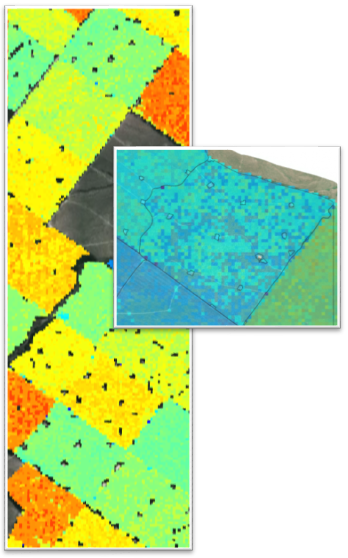+64 7 350 3209 +61 2 8011 3645 info@interpine.co.nz
Select Page

A team of researchers at Interpine recently completed a case study where stand attributes were derived from field measurements and LiDAR data using an approach known as nearest neighbor (kNN) imputation. The study was highly successful and produced unbiased and accurate estimates of key stand attributes across two forests in New Zealand’s North Island. Having calculated the average for a response variable for key forest attributes, a potential user of this approach will be interested in knowing:1. How does one calculate the sampling error of the kNN estimate for the Area of Internest (AOI) ?
2. How big/small is that sampling error relative to the alternatives?
3. What are the practical issues in calculating sampling error?
Calculating sampling error was one of the key technical challenges of the case studies and the calculation of sampling error estimates of this type is the subject of on-going statistical research. Spatial correlation is the tendency for plots that are physically close together to be similar. If not adequately accounted for spatial correlation in the reference dataset used to calibrate the kNN model can lead to inaccurate estimates of sampling error. The spatial correlation in the case study datasets were explored and used in the calculation of sampling errors for all stands in both study areas. This methodology followed was originally developed by Ron McRoberts and his colleagues at the U.S.D.A. (McRoberts et al. 2007) and as the process was computationally demanding a sampling technique were also successfully used.  Critically the sampling error for any kNN forest parameter can now be calculated for any area of interest in the study area.
The imputed stand parameters were compared to pre-existing and independent traditional forest inventory values for stands in the study area. The estimates of stand parameters produced by kNN imputation and traditional forest inventory were very similar in most cases. The kNN estimates of sampling error were generally smaller than those produced from the traditional inventory measurement. In one case study site the median kNN confidence interval for stands in the validation dataset was 27.9m3/ha compared to 37.89m3/ha from the traditional inventory. This result highlights the ability of the kNN approach to provide accurate and precise estimates of stand parameters for many stands from a much small number of field plots than are generally required for a traditional forest inventory.